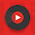## Solved Objective Questions from Basic Electrical Engineering

 At resonance, the RLC series circuit behaves as a
A. Purely resistive circuit
B. Purely inductive circuit
C. Capacitive circuit
D. Reactive Circuit

 The demand factor for the electrical system is the ratio of
A. Maximum demand to connected load
B. Maximum demand to average load
C. Average power to maximum power
D. Relative power to total power

 When a low resistance is connected in parallel with a high resistance, the combined resistance is
A. Always more than the high resistance
B. Always less than the low resistance
C. Always between the high resistance & low resistance
D. Either lower of higher than low resistance depending on the value of high resistance

 In RLC circuit, the phase of current with respect to the circuit voltage will be
B. Same
C. Lagging
D. Depends on L & C values

 The superposition theorem is based on the concept of
A. Duality
B. Linearity
C. Reciprocity
D. Non - Linearity

 Which of the following is same is all part of the series circuit?
A. Voltage
B. Current
C. Power
D. Resistance

 An electric machine will have high efficiency when
A. Input/output ratio is low
B. Reactive power is more
C. KWh consumption is low
D. Losses are low

 In a PNP circuit, the collector
A. Has a arrow pointing inward
B. Is positive with respect to the emitter
C. Is biased at a small fraction of the base bias
D. Is negative with respect to the emitter

 A PNP transistor can be replaced with an NPN device and the circuit will do the same thing, provided that
A. The power supply or battery polarity is reversed
B. The collector and emitter leads are interchanged
C. The arrow is pointing inward
D. A PNP transistor can never be replaced with NPN transistor

 The form factor of a ac voltage is
A.  RMS value/ Average value
B.  Average Value/ RMS Value
C.  Instantaneous Value/ Average value
D.  Average value/ Instantaneous value

5:56 PM

1.You have posted a good preparation material for electrical engineers, I like it..

2.1.3.